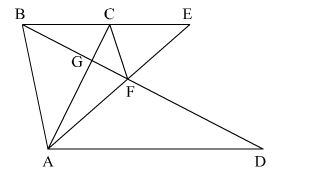# In the adjoining figure, CE || AD and CF || BA.

Question:

In the adjoining figure, CE || AD and CF || BA. Prove that ar(∆CBG) = ar(∆AFG).Solution:

$\operatorname{ar}(\triangle \mathrm{CFA})=\operatorname{ar}(\triangle \mathrm{CFB})$   (Triangles on the same base CF and between the same parallels CF || BA will be equal in area)

$\Rightarrow \operatorname{ar}(\triangle$ CFA $)-\operatorname{ar}(\triangle$ CFG $)=\operatorname{ar}(\triangle$ CFB $)-\operatorname{ar}(\triangle$ CFG $)$

$\Rightarrow \operatorname{ar}(\triangle \mathrm{AFG})=\operatorname{ar}(\triangle \mathrm{CBG})$

Hence Proved